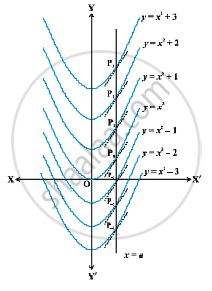# Geometrical Interpretation of Indefinite Integral

#### notes

Let f (x) = 2x. Then ∫f(x)dx  = x^2 +C.
y = x^2 +C  where C is arbitrary constant, represents a family of integrals. By assigning different values to C, we get different members of the family. These together constitute the indefinite integral. In this case, each integral represents a parabola with its axis along y-axis.
Clearly, for C = 0, we obtain y = x^2, a parabola with its vertex on the origin. The curve y = x^2 + 1 for C = 1 is obtained by shifting the parabola y = x^2 one unit along y-axis in positive direction.
For C = – 1, y = x^2 – 1 is obtained by shifting the parabola y = x^2 one unit along y-axis in the negative direction. Thus, for each positive value of C, each parabola of the family has its vertex on the positive side of the y-axis and for negative values of C, each has its vertex along the negative side of the y-axis. Fig.Let us consider the intersection of all these parabolas by a line x = a. In the above Fig.  we have taken a > 0. The same is true when a < 0. If the line x = a intersects the parabolas y =x^2 , y=x^2+1 , y =x^2+2, y = x^2-1 , y = x^2-2  at  P_0, P_1, P_2, P_(–1), P_(–2) etc., respectively, then (dy)/(dx)  at these points equals 2a. This indicates that the tangents to the curves at these points are parallel.
Thus ,∫2x dx = x^2 + C = F_C (x) (say), implies that the tangents to all the curves y = F_C (x), C ∈ R, at the points of intersection of the curves by the line x = a, (a ∈ R), are parallel.
Further, the following equation (statement)
∫ f(x) dx = F(x) +C = y  (say) ,
represents a family of curves. The different values of C will correspond to different members of this family and these members can be obtained by shifting any one of the curves parallel to itself. This is the geometrical interpretation of indefinite integral.

If you would like to contribute notes or other learning material, please submit them using the button below.

### Shaalaa.com

Integrals part 4 (Geometrical interpretion indefinite integrals) [00:03:30]
S
0%